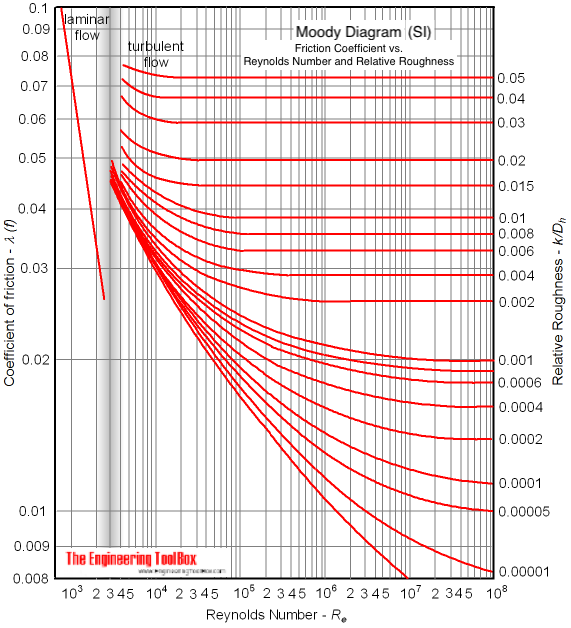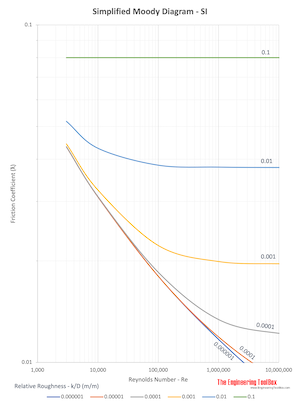Engineering ToolBox - Resources, Tools and Basic Information for Engineering and Design of Technical Applications!

# Colebrook Equation

## Friction loss coefficients in pipes, tubes and ducts.

The friction coefficients used when calculating resistance or pressure loss (or major loss) in ducts, tubes or pipes can be calculated with the Colebrook equation

1 / λ1/2 = -2 log [ 2.51 / (Re λ1/2)  +  (k / dh) / 3.72 ]                           (1)

where

λ = Darcy-Weisbach  friction coefficient

Re = Reynolds Number

k = roughness of duct, pipe or tube surface (m, ft)

dh = hydraulic diameter (m, ft)

The Colebrook equation is only valid at turbulent flow conditions.

Note! - the friction coefficient is involved on both sides of the equation. The equation must therefore be solved by iteration.

The Colebrook equation is generic and can be used to calculate friction coefficients for different kinds of fluid flows - like air ventilation ducts, pipes and tubes with water or oil, compressed air and much more.

### Colebrook Online Calculator

The friction coefficient can be calculated with the online Colebrook calculator below.

The default values used are for turbulent air flow in a 315 mm duct with 6 m/s (0.47 m3/s). The kinematic viscosity used to calculate the Reynolds Number is 15 10-6 m2/s.

hydraulic diameter - dh - (m, ft)

roughness of surface - k - (m, ft)

Reynolds Number - Re

Replace the parameters with the appropriate values for your application.

The calculator below can be used to calculate the friction coefficient if velocity and kinematic viscosity of the fluid are known.

hydraulic diameter - dh - (m, ft)

roughness of surface - k - (m, ft)

velocity - u - (m/s, ft/s)

kinematic viscosity - ν - (m2/s, ft2/s)

The friction coefficient can also be estimated with the Moody diagram (SI based):### Simplified Moody Diagram - SI## Related Topics

• Fluid Mechanics - The study of fluids - liquids and gases. Involving velocity, pressure, density and temperature as functions of space and time.
• Ventilation - Systems for ventilation and air handling - air change rates, ducts and pressure drops, charts and diagrams and more.

## Engineering ToolBox - SketchUp Extension - Online 3D modeling!

Add standard and customized parametric components - like flange beams, lumbers, piping, stairs and more - to your Sketchup model with the Engineering ToolBox - SketchUp Extension - enabled for use with the amazing, fun and free SketchUp Make and SketchUp Pro .Add the Engineering ToolBox extension to your SketchUp from the SketchUp Pro Sketchup Extension Warehouse!

Translate

## Privacy

We don't collect information from our users. Only emails and answers are saved in our archive. Cookies are only used in the browser to improve user experience.

Some of our calculators and applications let you save application data to your local computer. These applications will - due to browser restrictions - send data between your browser and our server. We don't save this data.

## Citation

• Engineering ToolBox, (2008). Colebrook Equation. [online] Available at: https://www.engineeringtoolbox.com/colebrook-equation-d_1031.html [Accessed Day Mo. Year].

Modify access date.

. .

#### Scientific Online Calculator3 30